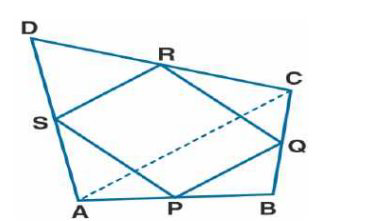# ML Aggarwal Solutions Class 9 Mathematics Solutions for Mid Point Theorem Exercise 11 in Chapter 11 - Mid Point Theorem

In the adjoining figure, ABCD is a quadrilateral in which P, Q, R and S are mid-points of AB, BC, CD

and DA respectively. AC is its diagonal. Show that

(i) SR || AC and SR = ½ AC

(ii) PQ = SR

(iii) PQRS is a parallelogram.It is given that

P, Q, R, and S are the mid-points of sides AB, BC, CD, and DA

AC is the diagonal

To find:

(i) SR || AC and SR = ½ AC

(ii) PQ = SR

(iii) PQRS is a parallelogram

Proof:

S and R are the mid-points of AD and DC

SR || AC and SR = ½ AC….. (1) Using the mid-point theorem

(ii) In Δ ABC

P and Q are the midpoints of AB and BC

PQ || AC and PQ = ½ AC ….. (2)

Using equation (1) and (2)

PQ = SR and PQ || SR

(iii) PQ = SR and PQ || SR

Hence, PQRS is a parallelogram.

Related Questions
Exercises

Lido

Courses

Teachers

Book a Demo with us

Syllabus

Maths
CBSE
Maths
ICSE
Science
CBSE

Science
ICSE
English
CBSE
English
ICSE
Coding

Terms & Policies

Selina Question Bank

Maths
Physics
Biology

Allied Question Bank

Chemistry
Connect with us on social media!